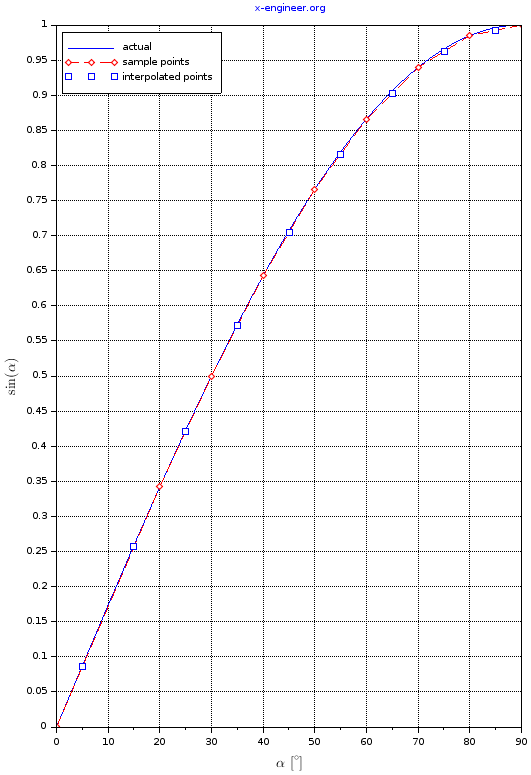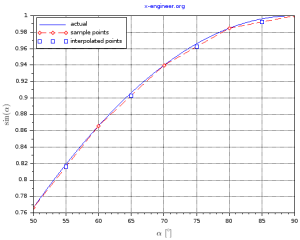# Linear interpolation and extrapolation with calculator

Linear interpolation is a mathematical method of using the equation of a line in order to find a new data point, based on an existing set of data points. Linear extrapolation is the same as linear interpolation, with the exception of the new data points, which are outside the range of the given (known) data points.

With other words, with linear interpolation and extrapolation, we can find new data points by approximating the current (known) data points as lines.

To apply linear interpolation or extrapolation, we need to know the coordinates of two points. These points will define the equation of a line, which will be used to find any new set of data points along the line.

For example, we know the coordinates of the points A (x1, y1) and B (x2, y2). What y will be for any given x ? To find the value of y, for a given, x1, y1, x2, y2 and x, we need to apply the linear interpolation (extrapolation) method.

Step 1. Calculate the slope m of the line, with the equation:

$m = \frac{y_{2}-y_{1}}{x_{2}-x_{1}} \tag{1}$

Step 2. Calculate the value of y using the line equation:

$y = y_{1} + m \cdot (x – x_{1}) \tag{2}$

For a better understanding, let’s look at some practical examples.

Example 1 (Linear interpolation). Given the points (1, 2) and (5, 7), calculate the value of y when x = 2.

Step 0. Extract the coordinates of the given data points.

$\begin{split} x_{1} = 1\\ y_{1} = 2\\ x_{2} = 5\\ y_{2} = 7 \end{split}$

Step 1. Calculate the slope of the line using equation (1):

$m = \frac{7-2}{5-1} = 1.25$

Step 2. Calculate the value of y using equation (2):

$y = 2 + 1.25 \cdot (2 – 1) = 3.25$

Since x is inside the interval [x1, x2], we performed a linear interpolation to find the value of y.

Example 2 (Linear extrapolation). Given the same points (1, 2) and (5, 7), calculate the value of y when x = -2.

Step 0 and 1 are the same as in the previous example, which allows us to calculate the value of y as:

$y = 2 + 1.25 \cdot (-2 – 1) = -1.75$

Since x is outside the interval [x1, x2], we performed a linear extrapolation to find the value of y.

### Linear interpolation and extrapolation online calculator

You can use the calculator below for linear interpolation and extrapolation, in order to calculate your own data points.

 x1 = x2 = y1 = y2 = x = y = 6 Interpolation

### Application of linear interpolation in embedded systems

One very common application of linear interpolation is in embedded systems. With a given set of data points, we can approximate different mathematical function and use linear interpolation to calculate the output of that function for a given input.

A function y(x) can be approximated on a fixed interval [x1, xN] by taking several sample points. Between these points, the linear interpolation method is applied to estimated the output y of the function for a given input x.

Let’s take as an example the trigonometrical function sin(α). It’s often the case that embedded applications do not have predefined trigonometrical functions but they are still used in internal calculations. One way to overcome this is to sample the trigonometrical function in different points and used linear interpolation to find its value for any given input.

Example. Replace the trigonometrical function sin(α), for α between 0° and 90°, with a set of data points, with an relative error less than 2%.

Step 1. Define the sample data points for the function:

 x = α [°] y = sin(α) x1 = 0 y1 = 0 x2 = 20 y2 = 0.3420201 x3 = 30 y3 = 0.5 x4 = 40 y4 = 0.6427876 x5 = 50 y5 = 0.7660444 x6 = 60 y6 = 0.8660254 x7 = 70 y7 = 0.9396926 x8 = 80 y8 = 0.9848078 x9 = 90 y9 = 1

Step 2. Define the interpolation function, which is going to use the sample data points and for any give angle, between 0 and 90, will return the sinus of the angle.

For this particular example, we are going to use Scilab for the definition of the interpolation function lininterp1d(axis, map, x). The linear interpolation function will have 3 arguments:

• axis: which is an array containing the xN points
• map: array containing the yN points
• x: point in which the function will be evaluated

The output of the function will be y, which is the value of the function in the x point.

function [y]=lininterp1d(axis,map,x)
if (x<=axis(1)) then y=map(1); elseif (x >= axis(length(axis))) then
y=map(length(axis));
else
for i=1:length(axis)
if (x==axis(i))
y=map(i);
break;
elseif ((x > axis(i)) && (x < axis(i + 1)))
x1 = axis(i);
x2 = axis(i + 1);
y1 = map(i);
y2 = map(i + 1);
slope = (y2 - y1) / (x2 - x1);
y = y1 + slope * (x - x1);
break;
end
end
end
endfunction


The above Scilab instructions will need to be saved in a file called lininterp1d.sci and loaded into the Scilab workspace before calling it.

Step 3. Evaluate the interpolation function for several input values and check the relative error.

Using a Scilab script, we are going to evaluate the interpolation function for the following angles α [°] (x values): 5, 15, 25, 35, 45, 55, 65, 75, 85.

// Sample points
xN_deg = [0 20 30 40 50 60 70 80 90];

// High resolution angle

// Evaluation points
x_deg = [5 15 25 35 45 55 65 75 85];

// Interpolation
for i=1:length(x_deg)
y(i) = lininterp1d(xN_deg,yN,x_deg(i));
end

// Plot
plot(alpha_deg,sin_alpha)
plot(xN_deg,yN,"dr--")
plot(x_deg,y,"sb")
title("x-engineer.org","Color","blue")
xgrid()
xlabel("$\alpha \text{ [} ^{\circ} \text{]}$","FontSize",3)
ylabel("$\text{sin}(\alpha)$","FontSize",3)
legend("actual","sample points","interpolated points",2)

// Display in Scilab console
clc()
mprintf("%s \t\t %s \t\t %s \t\t %s \n", "Interpolation", "Interpolated", "Real", "Relative");
mprintf("%s \t\t\t %s \t\t %s \t %s \n", "points", "output", "output", "error [%]");
for i=1:length(x_deg)
sin_x(i) = sin(x_deg(i)*%pi/180);
mprintf("%d \t\t\t %.6f \t\t %.6f \t %.6f\n", x_deg(i), y(i), sin_x(i), ((abs(sin_x(i)-y(i)))/y(i))*100);
end

// Predifined Scilab function for interpolation
[y]=interpln([xN_deg;yN],x_deg);
disp(y)


After running the Scilab instruction above, we’ll have displayed in the Scilab console:

Interpolation 		 Interpolated 		 Real 		 Relative
points 			 output 		 output 	 error [%]
5 			 0.085505 		 0.087156 	 1.930538
15 			 0.256515 		 0.258819 	 0.898168
25 			 0.421010 		 0.422618 	 0.381984
35 			 0.571394 		 0.573576 	 0.381984
45 			 0.704416 		 0.707107 	 0.381984
55 			 0.816035 		 0.819152 	 0.381984
65 			 0.902859 		 0.906308 	 0.381984
75 			 0.962250 		 0.965926 	 0.381984
85 			 0.992404 		 0.996195 	 0.381984


As you can see, the relative error of the interpolation is less than 2%. The same results are also plotted in the images below.Image: Linear interpolation based on Scilab datasetImage: Linear interpolation based on Scilab dataset – zoom in

For linear interpolation, Scilab has also its own predefined function:

[y]=interpln(xyd,x)

To get the same results, we can use the predefined function as:

[y]=interpln([xN_deg;yN],x_deg);

To try out the interpolation function algorithm based on datasets, we can use the online calculator below.

 xN = yN = x =

For more tutorials, click on the links below.